1. /
2. CBSE
3. /
4. Class 06
5. /
6. Mathematics
7. /
8. NCERT Solutions for Class...

# NCERT Solutions for Class 6 Maths Exercise 5.1

NCERT solutions for Maths Understanding elementary## NCERT Solutions for Class 6 Maths Understanding elementary

###### Question 1.What is the disadvantage in comparing line segments by mere observation?

Answer: There may be chance of error due to improper viewing.

###### Question 2.Why is it better to use a divider than a ruler, while measuring the length of a line segment?

Answer: It is better to use a divider than a ruler, because the thickness of the ruler may cause difficulties in reading off her length. However divider gives up accurate measurement.

NCERT Solutions for Class 6 Maths Exercise 5.1

###### Question 3.Draw any line segment, say {tex}\overline {{\text{AB}}} .{/tex} Take any point C lying in between A and B. Measure the lengths of AB, BC and AC. Is AB = AC + CB?
[Note: If A, B, C are any three points on a line, such that AC + CB = AB, hence we can be sure that C lies between A and B.]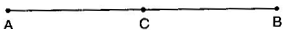AB = 6.5 cm, AC = 3cm, CB = 3.5 cm

AC + CB = 3 cm + 3.5 cm = 6.5 cm = AB

###### Question 4.If A, B, C are three points on a line such that AB = 5 cm, BC = 3cm and AC = 8 cm, which one of them lies between the other two?

Answer: {tex}\overline {{\text{AC}}} {/tex} is the longest line segment, thus B is the point between A and C.

NCERT Solutions for Class 6 Maths Exercise 5.1

###### Question 5.Verify whether D is the mid-point of {tex}\overline {{\text{AG}}} .{/tex}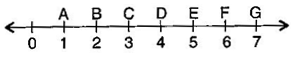Thus, D is the mid-point.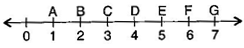###### Question 6. If B is the mid-point of {tex}\overline {{\text{AC}}} {/tex} and C is the mid-point of {tex}\overline {{\text{BD}}} ,{/tex} where A, B, C, D lie on a straight line, say why AB = CD?

Answer: B is the mid-point of {tex}\overline {{\text{AC}}} {/tex}.

{tex}\therefore {/tex} AB = BC ……….(i)

And C is the mid-point of {tex}\overline {{\text{BD}}} {/tex}.

{tex}\therefore {/tex} BC = CD ……….(ii)

From eq. (i) and (ii),

AB = CD

NCERT Solutions for Class 6 Maths Exercise 5.1

###### Question 7.Draw five triangles and measure their sides. Check in each case, of the sum of the lengths of any two sides is always less than the third side.

Answer: Yes, sum of two sides of a triangle is always greater than the third side.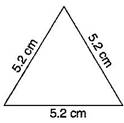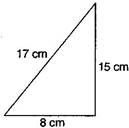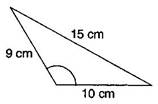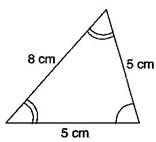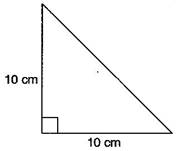## NCERT Solutions for Class 6 Maths Exercise 5.1

NCERT Solutions Class 6 Maths PDF (Download) Free from myCBSEguide app and myCBSEguide website. Ncert solution class 6 Maths includes text book solutions from Class 6 Maths Book . NCERT Solutions for CBSE Class 6 Maths have total 14 chapters. 6 Maths NCERT Solutions in PDF for free Download on our website. Ncert Maths class 6 solutions PDF and Maths ncert class 6 PDF solutions with latest modifications and as per the latest CBSE syllabus are only available in myCBSEguide.

## CBSE app for Students

To download NCERT Solutions for Class 6 Maths, Social Science Computer Science, Home Science, Hindi English, Maths Science do check myCBSEguide app or website. myCBSEguide provides sample papers with solution, test papers for chapter-wise practice, NCERT solutions, NCERT Exemplar solutions, quick revision notes for ready reference, CBSE guess papers and CBSE important question papers. Sample Paper all are made available through the best app for CBSE students and myCBSEguide website.Test Generator

Create question paper PDF and online tests with your own name & logo in minutes.myCBSEguide

Question Bank, Mock Tests, Exam Papers, NCERT Solutions, Sample Papers, Notes